Courses

# Test: Measurement of Power & Energy- 2

## 15 Questions MCQ Test Electrical and Electronic Measurements | Test: Measurement of Power & Energy- 2

Description
This mock test of Test: Measurement of Power & Energy- 2 for Electrical Engineering (EE) helps you for every Electrical Engineering (EE) entrance exam. This contains 15 Multiple Choice Questions for Electrical Engineering (EE) Test: Measurement of Power & Energy- 2 (mcq) to study with solutions a complete question bank. The solved questions answers in this Test: Measurement of Power & Energy- 2 quiz give you a good mix of easy questions and tough questions. Electrical Engineering (EE) students definitely take this Test: Measurement of Power & Energy- 2 exercise for a better result in the exam. You can find other Test: Measurement of Power & Energy- 2 extra questions, long questions & short questions for Electrical Engineering (EE) on EduRev as well by searching above.
QUESTION: 1

### A 230 V, single phase, watt hour meter has a constant load of 4 A passing through it for 6 hours at unity at unity power factor. The meter disc makes 2208 revolutions during this period. If the number of revolutions made by the meter are 1472 when operating at 230 V and 5 A for 4 hours, then power factor of the load would be

Solution:

Energy supplied = VI cosφ) x t x 10-3 kWh
= 230 x 4 x 1 x 6 x 10-3
= 5.52 kWh
Energy meter constant,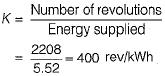Energy consumed when the meter makes 1472 revolutions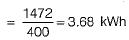Now, energy consumed
VI cos φ x f x 10-3 kWh = 230 x 5 x cost}) x 4 x 10-3 kWh
= 3.68 kWh (As obtained above)
or,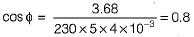Hence, power factor of the load = 0.8

QUESTION: 2

### The declared constant of a 5 A, 220 V d.c. watthour meter is 3275 revolutions per kWh. The speed of the disc at full load is

Solution:

Here, cos φ = 1 (for d.c. watthour meter) Energy supplied to the energy meter in kWh∴ Energy supplied to the energy meter per minute =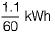Given, energy meter constant, K = 3275 revolutions/kWh
∴ Disc speed in rpm = Energy supplied per minute x Energy meter constant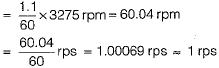QUESTION: 3

### In an induction type energy meter, an increase in temperature results in

Solution:

An increase in temperature is accompanied by a rise in resistance of ail copper and aluminium parts.

QUESTION: 4

A dynamometer wattmeter measures power in a 50 Hz, single phase circuit without error, at all power factors. The resistance of the voltage coil and its series resistance are 400 Ω and 10,000 Ω, respectively. The series resistance has a distributed self-capacitance equivalent to a shunt capacity of 20 pF.What is the self inductance of the pressure coil?

Solution:

Given, Rp = 400Ω,
RS = 10,000 Ω
CC = 20 pF
Self inductance of the pressure coil is given by
Lp = CC x RS2
= 20 x 10-12 x (104)2
= 20 x 10-4 = 2 mH

QUESTION: 5

Assertion (A): For measuring the power factor of the load in case of a two-wattmeter method, load may be balanced or unbalanced.
Reason (R): For measuring the power in a three- phase circuit, load may be balanced or unbalanced, source may be balanced or unbalanced but there must be three-phase load connected to three-phase source via three- phase wires.​

Solution:

Measurement of power factor with the help of two-wattmeter method can be done only if load is balanced. Hence, assertion is not true. Reason is a correct statement.

QUESTION: 6

The voltage and current in a circuit are given by, v(t) = 5 + 2 sin(ωt + 30°) + 10 sin(3ωt - 60°) and i(t) = 10 + 5 sinωt + 8 sin(2ωt-30°)
The total power consumed in the circuit is

Solution:

Total power consumed in the circuit
= Average active power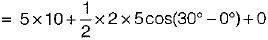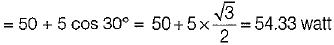QUESTION: 7

In an electrodynamometer type wattmeter
1. moving coil is iron cored.
2. fixed coil is air cored.
3. air friction damping is used.
4. the fixed coil is connected across the load.
5. the moving coil is connected in series with the load.

Q. Which of the above statements is/are true?

Solution:

In an electrodynamometer type wattmeter:
• Both fixed and moving coils are air cored.
• Air friction damping is used due to low value of operating magnetic field.
• The moving coil is connected across the load.
• The fixed coil is connected is series with the load.

QUESTION: 8

Assertion (A): A wattmeter must be used for the measurement of power in a.c. circuit instead of merely an ammeter and a voltmeter.
Reason (R): Wattmeter measures average active and reactive powers.

Solution:

A wattmeter must be used for the measurement of power in a.c. circuit instead of merely an ammeter and a voltmeter because the power factor can’t be measured with the help of voltmeter and ammeter.
Wattmeter measures average active power not average reactive power. Hence, reason is not a correct statement.

QUESTION: 9

Reason (R): The effect of pressure coil inductance is to increase the phase angle between load current and pressure coil current when the load power factor is leading.

Solution: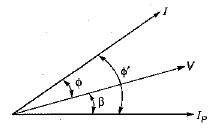In
In the above phasor diagram
IP = Pressure coil current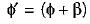We know that, measured power is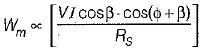QUESTION: 10

The ratio of measured power to true power in case of an electrodynamometer type wattmeter is given by (β = internal angle of pressure coil circuit)

Solution:

We have: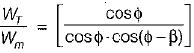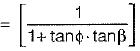or,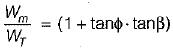QUESTION: 11

Assertion (A): Phantom loading of energy meter consists of supplying the fixed coil circuit from a circuit of required normal voltage, and the moving coil circuit from a separate low voltage supply.
Reason (R): When the current rating of a meter under test is high, a test with actual loading arrangement would involve a considerable waste of power due to which phantom or fictious loading is done.

Solution:

Phantom loading consists of supplying the pressure coil circuit (or moving coil circuit) from a circuit of required normal voltage, and the current circuit (or fixed coil circuit) from a separate low voltage supply. Hence, assertion is not true.
Reason is a correct statement.

QUESTION: 12

A dynamometer wattmeter with its voltage coil connected across the load side of the instrument reads 250 W, If the load voltage be 200 V, what power is being taken by the load?​
(The voltage coil has a resistance of 2000 Ω)

Solution: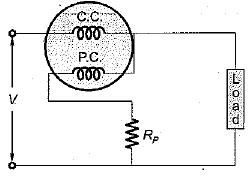Power loss in the voltage coil =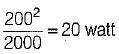∴ Actual load = 250 - 20
= 230 watt

QUESTION: 13

The power flowing in a 3- φ, 3-wire, balanced load system is measured by the two-wattmeter method. The reading on wattmeter -A is 3 kW and on wattmeter -B is-1 kW. The factor of the load is

Solution: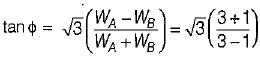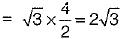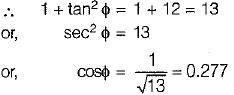QUESTION: 14

Holes are filled on the opposite side of the disc of an induction type energy meter to

Solution:

Cripping is due to over compensation provided to the running friction. To avoid it holes are made on opposite side of the disc of the energy meter.

QUESTION: 15

Two-wattmeter method is employed to measure power in a 3-phase balanced system with the current coils connected in the A and C lines. The phase sequence is ABC. If the wattmeter with its current coil in A-phase line reads zero, then the power factor of the 3-phase load will be

Solution:

When power factor = 0.5 (φ = 60°), then one wattmeter will read zero. The load will be lagging in nature.
W1 = VLIL cos (30 + φ)
and W2 = VLIL cos (30 - φ)
For φ = 60°, W1 = 0 watt